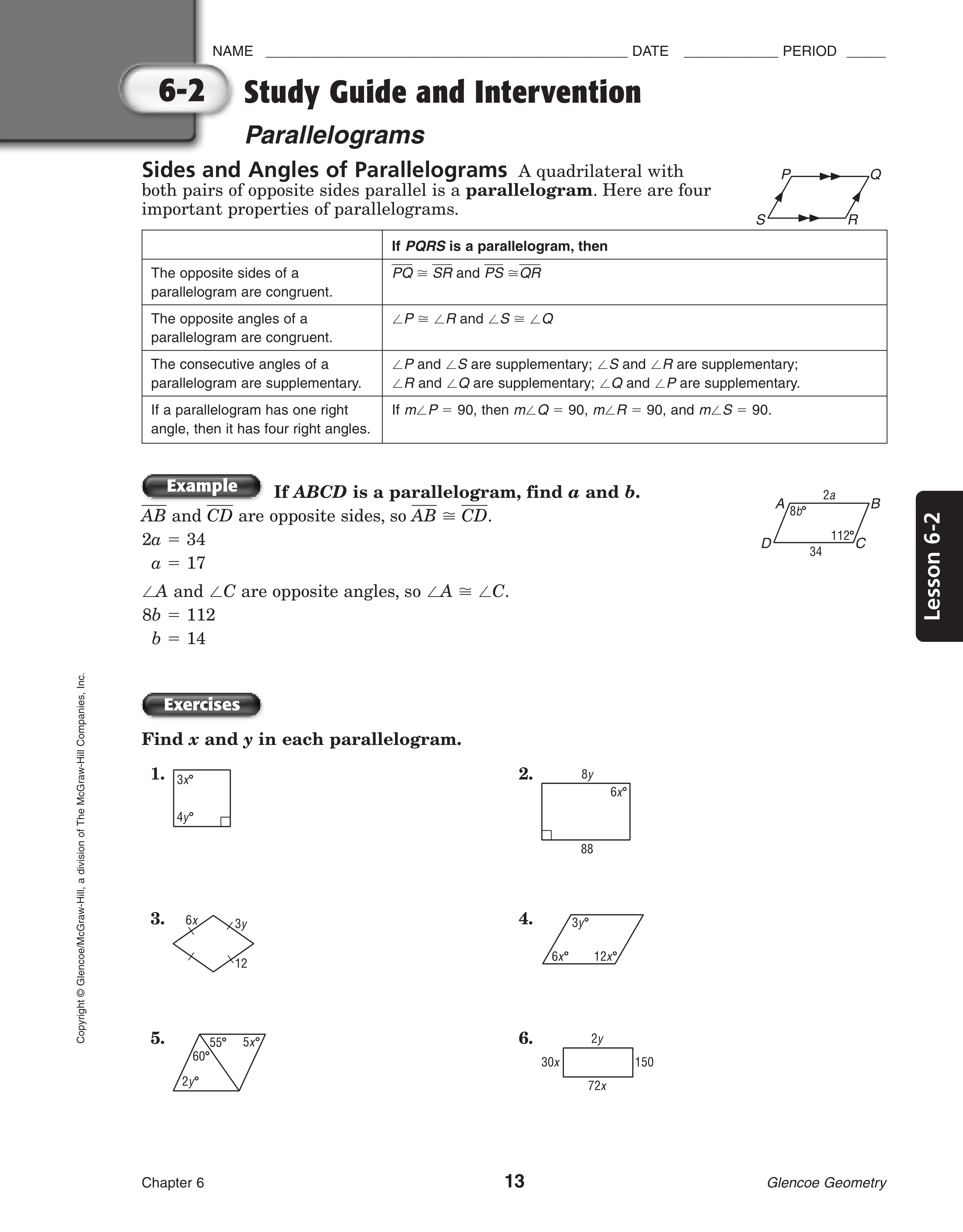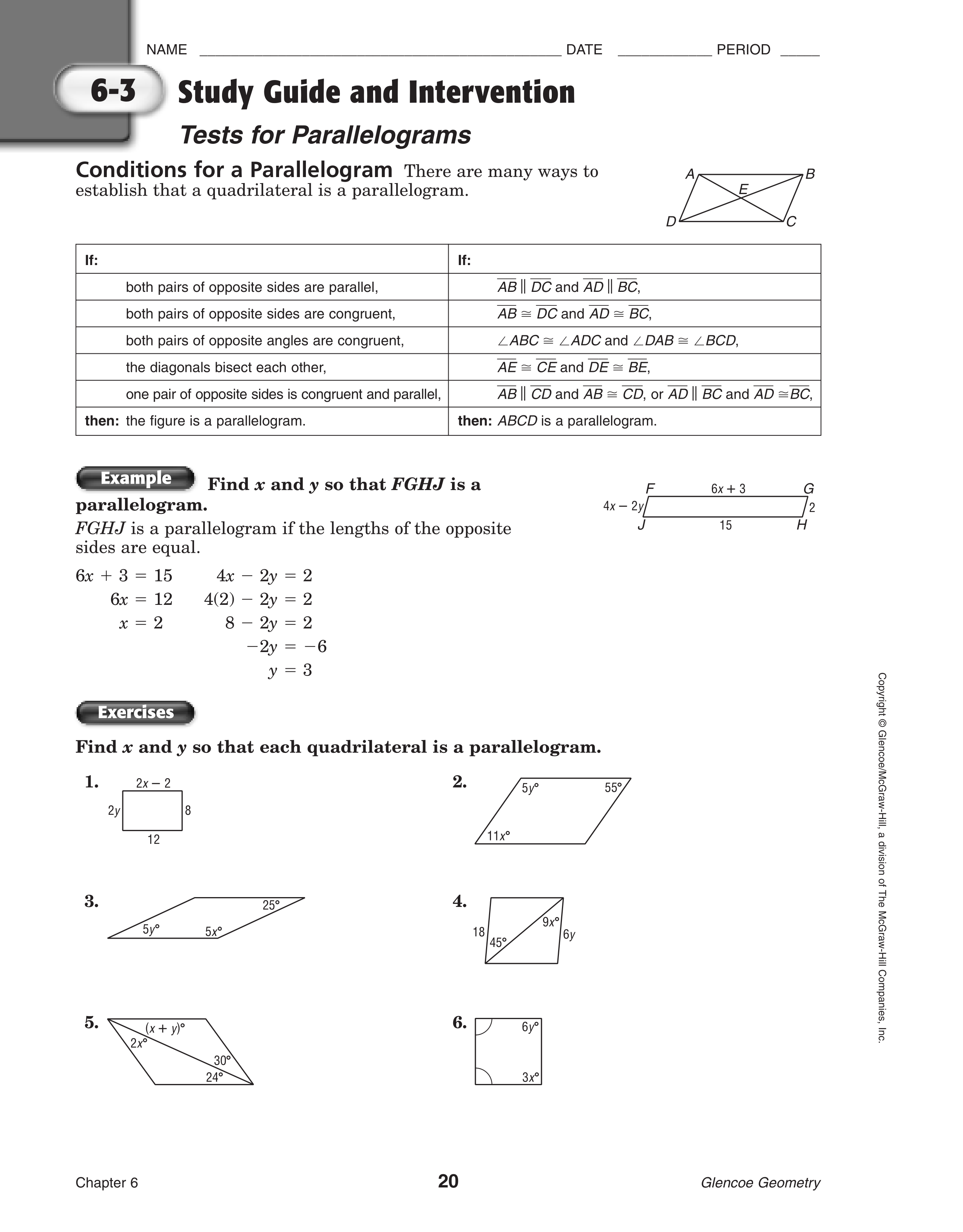Kindergarten
1st
2nd
3rd
4th
5th
6th
7th
8th
9th
10th
11th
12th
Higher Ed
Other
Subjects
ELA
Math
Science
Social Studies
Art
Computer Science
French
German
Music
Physical Education
Spanish
Other
Private Library
Geometry Parallelograms
starstarstarstarstarstarstarstarstarstar
by Matthew Rodolewicz
| 34 Questions
zoom in1
1 pt
x=
2
1 pt
y =
3
1 pt
x =
4
1 pt
y =
5
1 pt
x =
6
1 pt
y =
7
1 pt
x =
8
1 pt
y =
9
1 pt
x =
10
1 pt
y =
11
1 pt
x =
12
1 pt
y =
zoom in13
1 pt
x =
14
1 pt
y =
15
1 pt
x =
16
1 pt
y =
17
1 pt
x =
18
1 pt
y =
19
1 pt
3/10
10
10/3
20
1 pt
y =
21
1 pt
x =
22
1 pt
y =
zoom in23
1 pt
x =
24
1 pt
y =
25
1 pt
x =
26
1 pt
y =
27
1 pt
x =
28
1 pt
y =
29
1 pt
x =
30
1 pt
y =
31
1 pt
x =
32
1 pt
y =
33
1 pt
x =
34
1 pt
y =
zoom inAdd to my formatives list